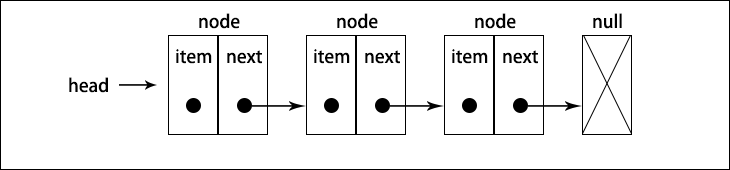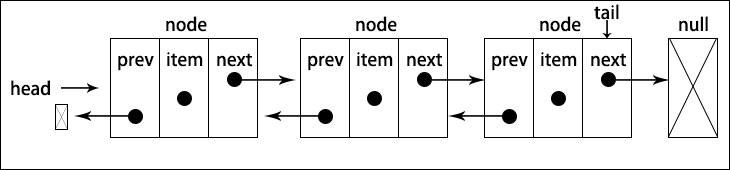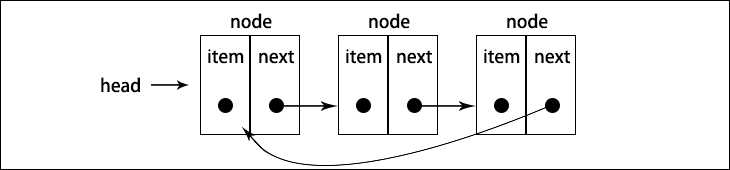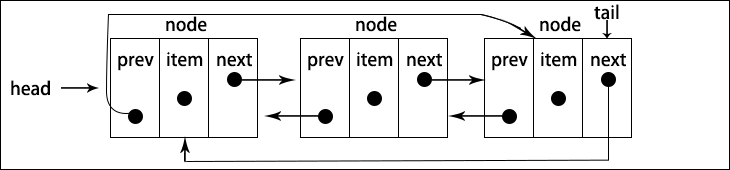# 为什么我要放弃javaScript数据结构与算法（第五章）—— 链表

• 链表数据结构
• 向链表添加元素
• 从链表移除元素
• 使用 LinkedList 类
• 双向链表
• 循环链表

## 第五章 链表

### 链表数据结构### 创建链表

function LinkedList(){
let Node = function(element){ // 需要一个Node辅助类，表示要加入列表的项，element 代表要添加到列表中的值， next d代表指向列表的下一个节点向的指针
this.element = element;
this.next = null;
}

let length = 0; // 存储列表项的数量 length 属性
let head = null; // 存储第一个节点的引用在 head 变量

this.append = function(element){};
this.insert = function(position,element){}
this.removeAt = function(position){}
this.remove = function(element){}
this.indexOf = function(position){}
this.isEmpty = function(){}
this.size = function(){}
this.getHead = function(){}
this.toString = function(){}
this.print = function(){}

}

LinkedList 类的方法的功能

• append(element)：向列表尾部添加一个新的项
• insert(position,element)：向列表的特定位置插入一个新的项
• removeAt(position)：从列表的特定位置移除一项
• remove(element)：从列表中移除一项
• indexOf(element)：返回元素在列表中的索引。如果列表中没有该元素则返回-1
• isEmpty()：如果链表中不包含任何元素，返回true,如果链表的长度大于0则返回 false
• size()：返回链表包含的元素个数，与数字的 length 属性类似
• toString()：由于列表项使用 Node 类，就需要重写继承自 JavaScript 对象默认的 toString 方法，让其只输出元素的值。

#### 向链表尾部追加元素

this.append = function(element){
let node = new Node(element),current;
if(head === null){
head = node;
}else{
current = head;
// 循环列表，直到找到最后一项
while(current.next){
current = current.next;
}
// 当current.next元素为null时，找到最后一项，将其 next 赋为 node,建立连接
current.next = node;
}
length++;  // 更新列表的长度
};

let list = new LinkedList();
console.log(list.append(15)); // Node?{element: 15, next: null}
console.log(list.append(10)); // Node?{element: 10, next: null}

#### 从链表中移除元素

this.removeAt = function(position){
// 检查越界值
if(position >-1 && position < length){
let current = head,previous,index = 0;

// 移除第一项
if(position === 0){
head = current.next;
console.log(current.element);
}else{
while(index++ < position){
previous = current;
current = current.next;
}
// 将 previous 与 current 的下一项连接起来，跳过 current,从移除它
previous.next = current.next;
}
length --;
console.log(current.element);
return current.element;
}else{
return null;
}
}

#### 在?#25105;?#20301;置插入元素

this.insert = function(position,element){
// 检查越界值
if(position >=0 && position <= length){
let node = new Node(element),
current = head,
previous,
index = 0;
if(position === 0 ){ // 在第一个位置添加
node.next = current;
head = node;
}else{
while(index++ < position){
previous = current;
current = current.next;
}
node.next = current;
previous.next = node;
}
length++;
return true;
}else{
return false;
}
}

current 变量是对列表中第一个元素的引用，我们需要做的是把 node.next 的值设为 current （列表中的第一个元素），现在 head 和 node.next 都指向了 current ，接下来要做的就是把 head 的引用改为 node ，这样列表中就有了一个新元素。

#### 实现其他方法

toString方法

this.toString = function(){
let current = head,
string = '';
while(current){
string += current.element + (current.next ? '-':'');
current = current.next;
}
return string;
}

indexOf方法

indexOf方法接受一个元素的值，如果在列表中找到它，就返回元素的位置，否则返回 -1

this.indexOf = function(element){
let current = head,
index = 0;
while(current){
if(element === current.element){
return index;
}
index++;
current = current.next;
}
return -1;
}

remove方法

this.remove = function(element){
let index = this.indexOf(element);
return this.removeAt(index);
}

isEmpty、size 和 getHead方法

isEmpty和size 和之前章节实现一模一样

this.isEmpty = function(){
return length === 0;
}

this.size = function(){
return length;
}

this.getHead = function(){
return head;
}

head 变量是 LinkedList 类的私有变量，我们如果需要在类的实现外部循环访问列表，就需要提供一种获取类的第一个元素的方法。

function LinkedList(){
let Node = function(element){ // 需要一个Node辅助类，表示要加入列表的项，element 代表要添加到列表中的值， next d代表指向列表的下一个节点向的指针
this.element = element;
this.next = null;
}

let length = 0; // 存储列表项的数量 length 属性
let head = null; // 存储第一个节点的引用在 head 变量

this.append = function(element){
let node = new Node(element),current;
if(head === null){
head = node;
}else{
current = head;
// 循环列表，直到找到最后一项
while(current.next){
current = current.next;
}
// 当current.next元素为null时，找到最后一项，将其 next 赋为 node,建立连接
current.next = node;
}
length++;  // 更新列表的长度
};

this.insert = function(position,element){
// 检查越界值
if(position >=0 && position <= length){
let node = new Node(element),
current = head,
previous,
index = 0;
if(position === 0 ){ // 在第一个位置添加
node.next = current;
head = node;
}else{
while(index++ < position){
previous = current;
current = current.next;
}
node.next = current;
previous.next = node;
}
length++;
return true;
}else{
return false;
}
}

this.removeAt = function(position){
// 检查越界值
if(position >-1 && position < length){
let current = head,previous,index = 0;

// 移除第一项
if(position === 0){
head = current.next;
console.log(current.element);
}else{
while(index++ < position){
previous = current;
current = current.next;
}
// 将 previous 与 current 的下一项连接起来，跳过 current,从移除它
previous.next = current.next;
}
length --;
return current.element;
}else{
return null;
}
}

this.remove = function(element){
let index = this.indexOf(element);
return this.removeAt(index);
}

this.indexOf = function(element){
let current = head,
index = 0;
while(current){
if(element === current.element){
return index;
}
index++;
current = current.next;
}
return -1;
}

this.isEmpty = function(){
return length === 0;
}

this.size = function(){
return length;
}

this.getHead = function(){
return head;
}

this.toString = function(){
let current = head,
string = '';
while(current){
string += current.element + (current.next ? '-':'');
current = current.next;
}
return string;
}

}

### 双向链表function DoublyLinkedList(){
let Node = function(elememt){
this.elememt = elememt;
this.next = null;
this.prev = null; // 新增的
}
let length = 0;
let head = null;
let tail = null // 新增的

// 这里是方法
}

#### 在?#25105;?#20301;置插入新元素

this.insert = function(position,elememt){
// 检查越界值
if(position >= 0 && position <= length){
let node = new Node(elememt),
current = head,
previous,
index = 0;
if(position === 0){ // 在第一个位置添加
if(!head){
head = node;
tail = node;
}else{
node.next = current;
current.prev = node;
head = node;
}
}else if(position === length){ // 最后一项
current = tail;
current.next = node;
node.prev = current;
tail = node;
}else{
while (index++ < position) {
previous = current;
current = current.next;
}
node.next = current;
previous.next = node;

current.prev = node;
node.prev = previous;
}
length++
return true;
}else{
return false;
}
}   

#### 从?#25105;?#20301;置移除元素

this.removeAt = function(position){
// 检查越界值
if(position > -1 && position < length){
let current = head,
previous,
index = 0;
// 移除第一项
if(position === 0){
head = current.next;
// 如果只有一项，更新 tail
if(length === 1){
tail = null;
}else{
head.prev = null;
}
}else if(position === length-1){ // 最后一项
current = tail;
tail = current.prev;
tail.next = null;
}else{
while (index++ < position) {
previous = current;
current = current.next;
}
// 将 previous 与 current 的下一项连接起来——跳过 current
previous.next = current.next;
current.next.prev = previous;
}
length --;
return current.elememt;
}else{
return null;
}
}

function DoublyLinkedList(){
let Node = function(elememt){
this.elememt = elememt;
this.next = null;
this.prev = null; // 新增的
}
let length = 0;
let head = null;
let tail = null // 新增的

this.append = function(elememt){
let node = new Node(elememt),
current;
if(!head){
head = node;
tail = node;
}else{
current = tail;
current.next = node;
node.prev = current;
tail = node;
}
length++;
}

this.insert = function(position,elememt){
// 检查越界值
if(position >= 0 && position <= length){
let node = new Node(elememt),
current = head,
previous,
index = 0;
if(position === 0){ // 在第一个位置添加
if(!head){
head = node;
tail = node;
}else{
node.next = current;
current.prev = node;
head = node;
}
}else if(position === length){ // 最后一项
current = tail;
current.next = node;
node.prev = current;
tail = node;
}else{
while (index++ < position) {
previous = current;
current = current.next;
}
node.next = current;
previous.next = node;

current.prev = node;
node.prev = previous;
}
length++
return true;
}else{
return false;
}
}

this.removeAt = function(position){
// 检查越界值
if(position > -1 && position < length){
let current = head,
previous,
index = 0;
// 移除第一项
if(position === 0){
head = current.next;
// 如果只有一项，更新 tail
if(length === 1){
tail = null;
}else{
head.prev = null;
}
}else if(position === length-1){ // 最后一项
current = tail;
tail = current.prev;
tail.next = null;
}else{
while (index++ < position) {
previous = current;
current = current.next;
}
// 将 previous 与 current 的下一项连接起来——跳过 current
previous.next = current.next;
current.next.prev = previous;
}
length --;
return current.elememt;
}else{
return null;
}
}

this.remove = function(elememt){
let index = this.indexOf(elememt);
this.removeAt(index);
}

this.toString = function(){
let current = head,
str = '';
while (current) {
str += current.elememt + (current.next?'-':'');
current = current.next;
}
return str;
}

this.indexOf = function(elememt){
let current = head,
index = 0;
while (current) {
if(current.elememt === elememt){
return index
}
current = current.next;
index++
}
return -1;
}

this.isEmpty = function(){
return length === 0;
}

this.size = function(){
return length;
}

this.getHead = function(){
return head;
}

}

### 循环链表### 小结

posted @ 2018-11-03 11:25 赖同学 阅读(...) 评论(...) 编辑 收藏